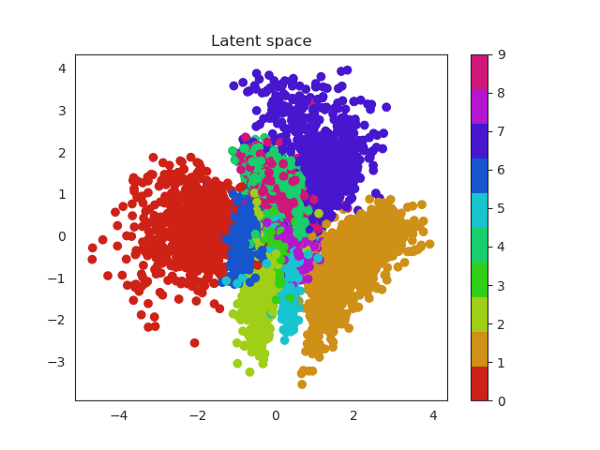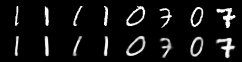×

Search anything:

# Generate new MNIST digits using Autoencoder

#### Machine Learning (ML) autoencoderGet this book -> Problems on Array: For Interviews and Competitive Programming

Reading time: 30 minutes | Coding time: 20 minutes

Autoencoder is a neural network tries to learn a particular feature of converting an input to an output data and generate back the input given the output. It includes two parts:

• encoder: which learns the features of the data or given answers
• decoder: which tries to generate the answers from the learnt features/ questions

This technique is widely used for a variety of situations such as generating new images, removing noise from images and many others.

In this article, we will learn how autoencoders can be used to generate the popular MNIST dataset and we can use the result to enhance the original dataset. We will build an autoencoder from scratch in TensorFlow and generate the actual images from the MNIST dataset.

## Idea of using an Autoencoder

The basic idea of using Autoencoders for generating MNIST digits is as follows:

• Encoder part of autoencoder will learn the features of MNIST digits by analyzing the actual dataset. For example, X is the actual MNIST digit and Y are the features of the digit. Our encoder part is a function F such that F(X) = Y.

• Decoder part of autoencoder will try to reverse process by generating the actual MNIST digits from the features. At this point, we have Y in F(X)=Y and try to generate the input X for which we will get the output.

The idea of doing this is to generate more handwritten digits dataset which we can use for a variety of situations like:

• Train a model better by covering a larger possibility of handwritten digits

As there will be multiple features of handwritten digits, until our autoencoder is over trained, we will generate a different set of handwritten digits than MNIST which is expected to differ by a small amount and will be beneficial in expanding the dataset.

## Building our Autoencoder

We use use TensorFlow's Python API to accomplish this.

Import all the libraries that we will need, namely tensorflow, keras, matplotlib, .

``````import tensorflow as tf
from tensorflow import keras
import matplotlib.pyplot as plt
``````

Define constant parameter for batch size (number of images we will process at a time).

``````batch_size = 128
``````

Fetch the data from MNIST dataset and load it. Note we take the MNIST dataset to learn the features which we will use to regenerate the dataset.

``````digits_mnist = keras.datasets.mnist
``````

Create local dataset using tensorflow. Train the data and split it into batches in accordance with the batch size. Reshape each of the image data to equivalent size.

``````with tf.variable_scope("DataPipe"):
dataset = tf.data.Dataset.from_tensor_slices(train_images)
dataset = dataset.map(lambda x: tf.image.convert_image_dtype([x], dtype=tf.float32))
dataset = dataset.batch(batch_size=batch_size).prefetch(batch_size)
iterator = dataset.make_initializable_iterator()
input_batch = iterator.get_next()
input_batch = tf.reshape(input_batch, shape=[-1, 28, 28, 1])
``````

Iterate through the batches till none are left

``````init_vars = [tf.local_variables_initializer(),tf.global_variables_initializer()]
with tf.Session() as sess:
sess.run([init_vars, iterator.initializer])
while 1:
try:
batch = sess.run(input_batch)
print(batch.shape)  # Get batch dimensions
plt.imshow(batch[0,:,:,0] , cmap='gray')
plt.show()
except tf.errors.OutOfRangeError:
print('All batches have been iterated!')
break
``````

## Encoding phase

This is the encoding function. Use convolutional layers along with padding to help maintain the spatial relations between pixels. Compute the mean, standard deviation and epsilon value. Calculate the value z using the first 3 values mentioned.

``````def encoder(X):
activation = tf.nn.relu
with tf.variable_scope("Encoder"):
x = tf.layers.conv2d(X, filters=64, kernel_size=4, strides=2, padding='same', activation=activation)
x = tf.layers.conv2d(x, filters=64, kernel_size=4, strides=2, padding='same', activation=activation)
x = tf.layers.conv2d(x, filters=64, kernel_size=4, strides=1, padding='same', activation=activation)
x = tf.layers.flatten(x)
mean_ = tf.layers.dense(x, units=FLAGS.latent_dim, name='mean')
std_dev = tf.nn.softplus(tf.layers.dense(x, units=FLAGS.latent_dim), name='std_dev')  # softplus to force >0
epsilon = tf.random_normal(tf.stack([tf.shape(x), FLAGS.latent_dim]), name='epsilon')
z = mean_ + tf.multiply(epsilon, std_dev)
return z, mean_, std_dev
``````

Note: Z captures the features of the MNIST dataset

## Decoding phase

This is the decoding function. Here, we transpose the convulations. But before that apply some non linear transformations using dense layers. To recover the original image, use the unsampling method from the latent variables.

``````def decoder(z):
activation = tf.nn.relu
with tf.variable_scope("Decoder"):
x = tf.layers.dense(z, units=FLAGS.inputs_decoder, activation=activation)
x = tf.layers.dense(x, units=FLAGS.inputs_decoder, activation=activation)
recovered_size = int(np.sqrt(FLAGS.inputs_decoder))
x = tf.reshape(x, [-1, recovered_size, recovered_size, 1])
x = tf.layers.conv2d_transpose(x, filters=64, kernel_size=4, strides=1, padding='same', activation=activation)
x = tf.layers.conv2d_transpose(x, filters=64, kernel_size=4, strides=1, padding='same', activation=activation)
x = tf.layers.conv2d_transpose(x, filters=64, kernel_size=4, strides=1, padding='same', activation=activation)
x = tf.contrib.layers.flatten(x)
x = tf.layers.dense(x, units=28 * 28, activation=None)
x = tf.layers.dense(x, units=28 * 28, activation=tf.nn.sigmoid)
img = tf.reshape(x, shape=[-1, 28, 28, 1])
return img
``````

## Running the encoding and decoding phase

``````z, mean_, std_dev = encoder(input_batch)
output = decoder(z)
``````

Reshape input and output to flat vectors.

``````flat_output = tf.reshape(output, [-1, 28 * 28])
flat_input = tf.reshape(input_batch, [-1, 28 * 28])
``````

Compute the loss function using the binary cross entropy formula. Then calculate the latent loss using the KL divergence formula and finally get the mean of all the image losses.

``````with tf.name_scope('loss'):
img_loss = tf.reduce_sum(flat_input * -tf.log(flat_output) + (1 - flat_input) * -tf.log(1 - flat_output), 1)
latent_loss = 0.5 * tf.reduce_sum(tf.square(mean_) + tf.square(std_dev) - tf.log(tf.square(std_dev)) - 1, 1)
loss = tf.reduce_mean(img_loss + latent_loss)
``````

## Train the model

This is the training loop. For each sample, we create an artificial image and display it. Latent space plot is also being created here

``````while True:
try:
sess.run(optimizer)
if flag:
summ, target, output_ = sess.run([merged_summary_op, input_batch, output])
f, axarr = plt.subplots(FLAGS.test_image_number, 2)
for j in range(FLAGS.test_image_number):
for pos, im in enumerate([target, output_]):
axarr[j, pos].imshow(im[j].reshape((28, 28)), cmap='gray')
axarr[j, pos].axis('off')

plt.savefig(os.path.join(results_folder, 'Train/Epoch_{}').format(epoch))
plt.close(f)
flag = False
artificial_image = sess.run(output, feed_dict={z: np.random.normal(0, 1, (1, FLAGS.latent_dim))})
plt.figure()
with sns.axes_style("white"):
plt.imshow(artificial_image.reshape((28, 28)), cmap='gray')
plt.savefig(os.path.join(results_folder, 'Test/{}'.format(epoch)))
plt.close()

if FLAGS.latent_dim == 2 and FLAGS.plot_latent:
coords = sess.run(z, feed_dict={input_batch: test_images[..., np.newaxis]/255.})
colormap = ListedColormap(sns.color_palette(sns.hls_palette(10, l=.45 , s=.8)).as_hex())
plt.scatter(coords[:, 0], coords[:, 1], c=test_labels, cmap=colormap)
cbar = plt.colorbar()
if FLAGS.dataset == 'digits-mnist':
cbar.ax.set_yticklabels(['one', 'two', 'three', 'four', 'five', 'six', 'seven', 'eight', 'nine'])

plt.title('Latent space')
plt.savefig(os.path.join(results_folder, 'Test/Latent_{}'.format(epoch)))
plt.close()

except tf.errors.OutOfRangeError:
break
``````

This is the plot of the latent space:Latent space is the output of the encoder phase which the decoder phase will use. Each point represents a feature or an input MNIST digit and the clusters represent that the points belong to a single digits.

The decoder phase uses this representation to regenerate the MNIST dataset.

Create a mesh grid of values. The matrix that will contain the grid of images.

``````values = np.arange(-3, 4, .5)
xx, yy = np.meshgrid(values, values)
input_holder = np.zeros((1, 2))
container = np.zeros((28 * len(values), 28 * len(values)))
``````

Run the test images in the matrix which generates the output.

``````for row in range(xx.shape):
for col in range(xx.shape):
input_holder[0, :] = [xx[row, col], yy[row, col]]
artificial_image = sess.run(output, feed_dict={z: input_holder})
container[row * 28: (row + 1) * 28, col * 28: (col + 1) * 28] = np.squeeze(artificial_image)

plt.imshow(container, cmap='gray')
plt.savefig(os.path.join(results_folder, 'Test/Space_{}'.format(epoch)))
plt.close()
``````

## Output

The top line is the input and bottom line is the output-The output image may seem to be similar to the input image but there are small differences which can be measured using a similarity metric.

Enjoy the autoencoder model#### Abhinav Prakash

Intern at 1LearnApp, Hoopstop, Harvesting and OpenGenus | Bachelor's degree (2016 to 2020) in Computer Science at University of Massachusetts, Amherst

Generate new MNIST digits using Autoencoder